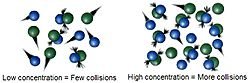# Chemical kinetics facts for kids

Kids Encyclopedia FactsWith a higher concentration, molecules will hit each other more easily and so the speed of the reaction will be bigger

Chemical kinetics, also called reaction kinetics, is studying how fast chemical reactions go. This includes studying how different conditions such as temperature, pressure or solvent used affect the speed of a reaction. Chemical kinetics can also be used to find out about reaction mechanisms and transition states.

The basic idea of chemical kinetics is called collision theory. This states that for a reaction to happen, the molecules must hit each other. Ways of increasing the speed of the reaction must therefore increase the number of hits. This can be done in many ways.

With experiments it is possible to calculate reaction rates from which you can get rate laws and rate constants. A rate law is a mathematical expression with which you can calculate the speed of a reaction given the concentration of the reagents.

## Order of a reaction

the equilibrium is dynamic in nature

There are many types of rate laws, but the most common are:

• zero-order reaction: the speed does not depend on the concentration
• first-order reaction: the speed depends on the concentration of only one reactant
• second-order reaction: the speed depends on the concentration of two reactants, or on the concentration of one reactant squared.

From this data, it is possible to think about the mechanism of the reaction. If it is second-order, for example, then it is likely that both molecules in the reaction are coming together during the rate-determining step. This is the most difficult step in the mechanism to go through, because it has the highest activation energy.Chemical kinetics Facts for Kids. Kiddle Encyclopedia.